### AUTOREG Statement

AUTOREG <options> ;

The AUTOREG statement specifies an autoregressive component in the model. An autoregressive component is a special case of cycle that corresponds to the frequency of zero or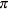. It is modeled separately for easier interpretation. A stochastic equation for an autoregressive component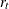can be written as follows: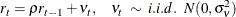The damping factor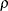can take any value in the interval (–1, 1), including –1 but excluding 1. If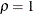, the autoregressive component cannot be distinguished from the random walk level component. If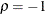, the autoregressive component corresponds to a seasonal component with a season length of 2, or a nonstationary cycle with period 2. If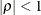, then the autoregressive component is stationary. The following example illustrates the AUTOREG statement. This statement includes an autoregressive component in the model. The damping factorand the disturbance variance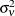are estimated from the data.

      autoreg;


NOEST=RHO
NOEST=VARIANCE
NOEST=(RHO VARIANCE)

fixes the values ofandto those specified in the RHO= and VARIANCE= options.

PLOT=FILTER
PLOT=SMOOTH
PLOT=( <FILTER> <SMOOTH> )

requests plotting of the filtered or smoothed estimate of the autoreg component.

PRINT=FILTER
PRINT=SMOOTH
PRINT=(<FILTER> <SMOOTH>)

requests printing of the filtered or smoothed estimate of the autoreg component.

RHO=value

specifies an initial value for the damping factorduring the parameter estimation process. The value ofmust be in the interval (–1, 1), including –1 but excluding 1.

VARIANCE=value

specifies an initial value for the disturbance varianceduring the parameter estimation process. Any nonnegative value, including zero, is an acceptable starting value.